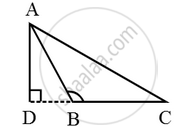# In Fig., ∆ABC is an obtuse triangle, obtuse angled at B. If AD ⊥ CB, prove that AC^2 = AB^2 + BC^2 + 2BC × BD - Mathematics

Sum

In Fig., ∆ABC is an obtuse triangle, obtuse angled at B. If AD ⊥ CB, prove that AC2 = AB2 + BC2 + 2BC × BD

#### Solution

Given: An obtuse triangle ABC, obtuse-angled at B and AD is perpendicular to CB produced.

To Prove:  AC2 = AB2 + BC2 + 2BC × BD

Proof: Since ∆ADB is a right triangle right angled at D. Therefore, by

Pythagoras theorem, we have AB2 = AD2 + DB2 ….(i)Again ∆ADC is a right triangle right angled at D.

Therefore, by Phythagoras theorem, we have

⇒ AC2 = AD2 + (DB + BC)2

⇒ AC2 = AD2 + DB2 + BC2 + 2BC • BD

⇒ AC2 = AB2 + BC2 + 2BC • BD [Using (i)]

Hence, AC2 = AB2 + BC2 + 2BC • BD

Concept: Right-angled Triangles and Pythagoras Property
Is there an error in this question or solution?